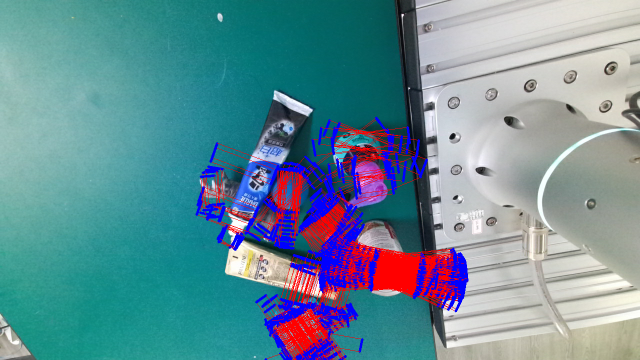# Convert Labels between rectangle format and 6d format¶

Get a GraspNet instance.

```from graspnetAPI import GraspNet
import cv2
import open3d as o3d

# GraspNetAPI example for checking the data completeness.
# change the graspnet_root path

camera = 'kinect'
sceneId = 5
annId = 3

####################################################################
graspnet_root = '/home/gmh/graspnet' # ROOT PATH FOR GRASPNET
####################################################################

g = GraspNet(graspnet_root, camera = camera, split = 'all')

bgr = g.loadBGR(sceneId = sceneId, camera = camera, annId = annId)
depth = g.loadDepth(sceneId = sceneId, camera = camera, annId = annId)
```

## Convert rectangle format to 6d format¶

First, load rectangle labels from dataset.

```# Rect to 6d
rect_grasp_group = g.loadGrasp(sceneId = sceneId, camera = camera, annId = annId, fric_coef_thresh = 0.2, format = 'rect')
```

Convert a single RectGrasp to Grasp.

Note

This conversion may fail due to invalid depth information.

```# RectGrasp to Grasp
rect_grasp = rect_grasp_group.random_sample(1)
img = rect_grasp.to_opencv_image(bgr)

cv2.imshow('rect grasp', img)
cv2.waitKey(0)
cv2.destroyAllWindows()

grasp = rect_grasp.to_grasp(camera, depth)
if grasp is not None:
geometry = []
geometry.append(grasp.to_open3d_geometry())
o3d.visualization.draw_geometries(geometry)
else:
print('No result because the depth is invalid, please try again!')
```

Before Conversion: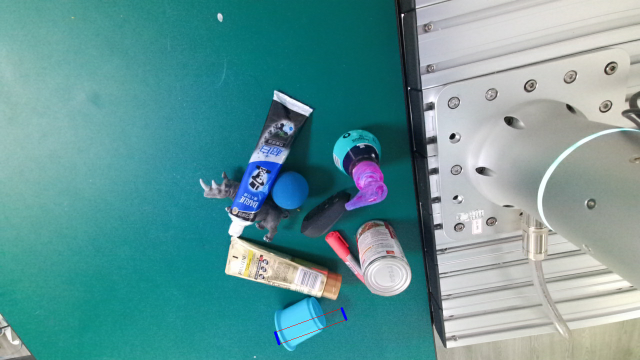After Conversion: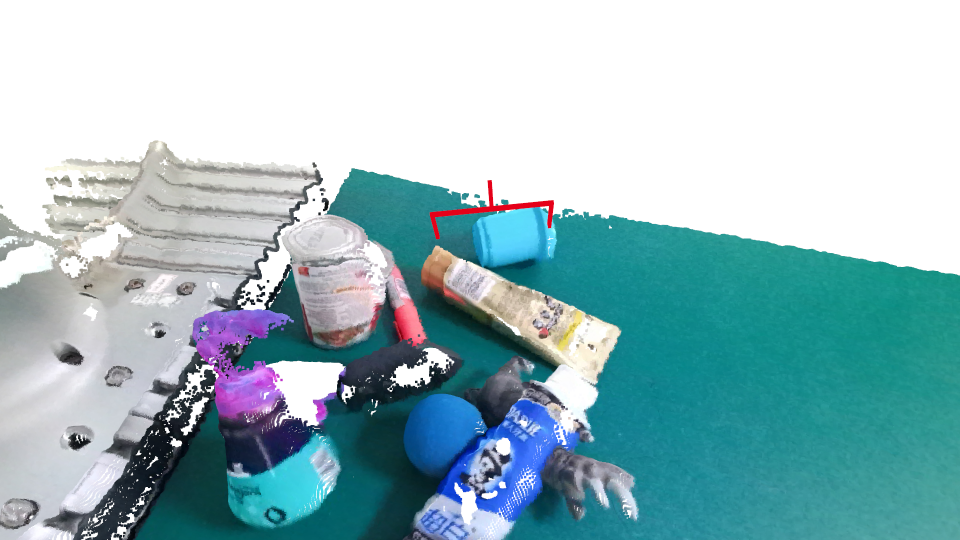Convert RectGraspGroup to GraspGroup.

```# RectGraspGroup to GraspGroup
sample_rect_grasp_group = rect_grasp_group.random_sample(20)
img = sample_rect_grasp_group.to_opencv_image(bgr)
cv2.imshow('rect grasp', img)
cv2.waitKey(0)
cv2.destroyAllWindows()

grasp_group = sample_rect_grasp_group.to_grasp_group(camera, depth)
if grasp_group is not None:
geometry = []
geometry += grasp_group.to_open3d_geometry_list()
o3d.visualization.draw_geometries(geometry)
```

Before Conversion: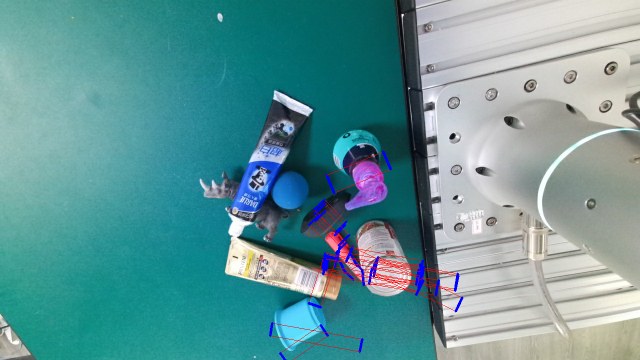After Conversion: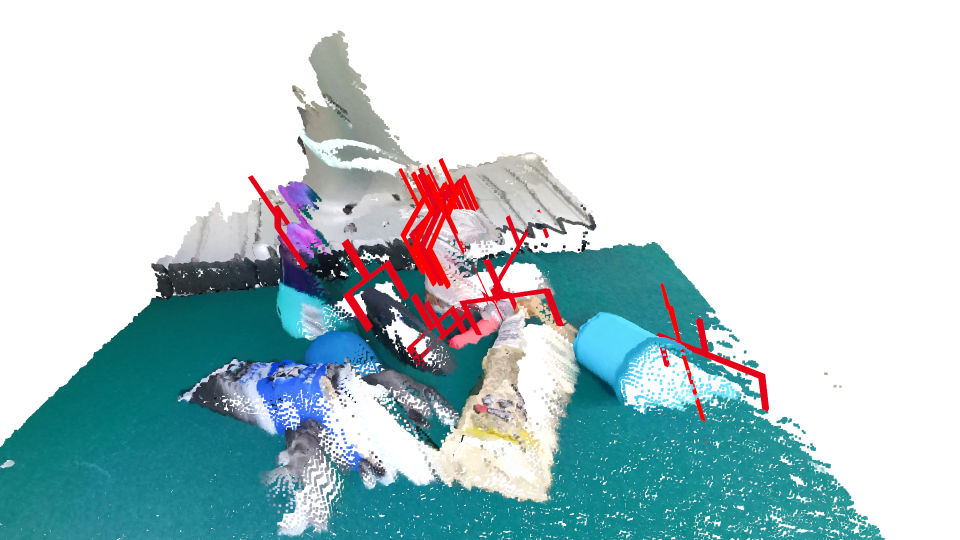## Convert 6d format to rectangle format¶

Note

Grasp to RectGrasp conversion is not applicable as only very few 6d grasp can be converted to rectangle grasp.

```# 6d to Rect
_6d_grasp_group = g.loadGrasp(sceneId = sceneId, camera = camera, annId = annId, fric_coef_thresh = 0.2, format = '6d')

# Grasp to RectGrasp conversion is not applicable as only very few 6d grasp can be converted to rectangle grasp.

# GraspGroup to RectGraspGroup
sample_6d_grasp_group = _6d_grasp_group.random_sample(20)
geometry = []
geometry += sample_6d_grasp_group.to_open3d_geometry_list()
o3d.visualization.draw_geometries(geometry)

rect_grasp_group = _6d_grasp_group.to_rect_grasp_group(camera)
img = rect_grasp_group.to_opencv_image(bgr)

cv2.imshow('rect grasps', img)
cv2.waitKey(0)
cv2.destroyAllWindows()

```

Before Conversion: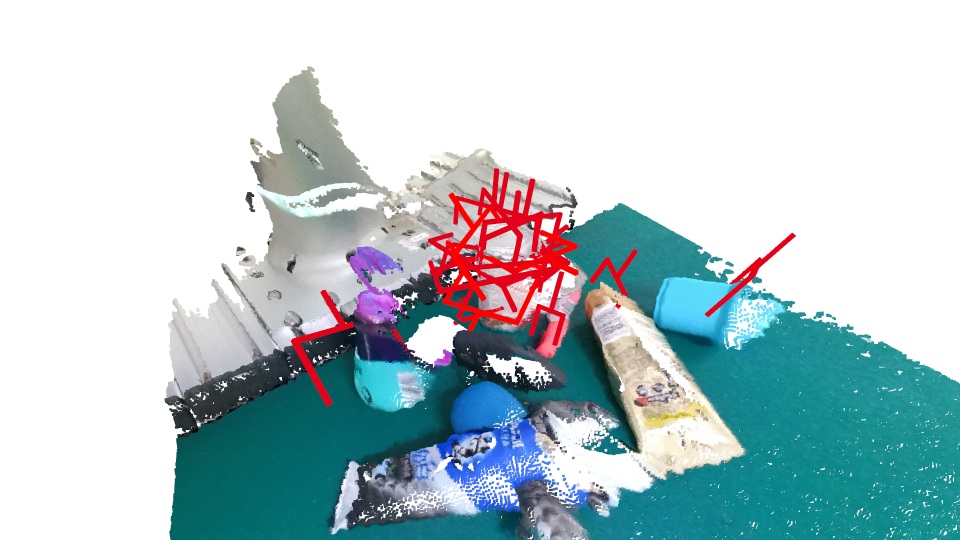After Conversion: